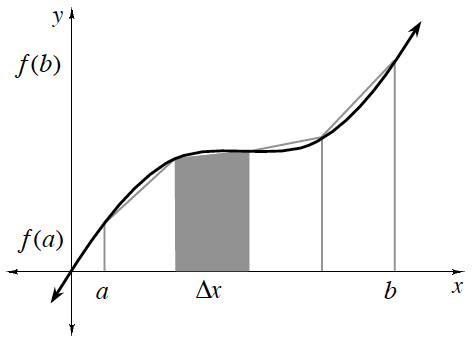### Home > APCALC > Chapter 2 > Lesson 2.4.1 > Problem2-137

2-137.

Write a single expression using the Trapezoid Rule, which will approximate the area under $f(x) = 2x^2 - 4x + 3$ over the interval $0\le x\le1$ using five trapezoids of equal height. Do not evaluate the expression.

#### The Trapezoid RuleThe Trapezoid Rule can be used to approximate the area under a curve if the widths, $\Delta x$, of all trapezoids are of equal size:

$\left. \begin{array} { r } { A = \frac { \Delta x } { 2 } ( f ( a ) + 2 f ( a + \Delta x ) + 2 f ( a + 2 \Delta x ) + \ldots } \\ { + 2 f ( b - \Delta x ) + f ( b ) ) } \end{array} \right.$

$\text{You know that you're writing an expression between }0\leq x\leq 1 \text{ using }5 \text{ trapezoids,}$

$\text{so you know that you'll be starting with }0 \text{ and increasing }x-\text{values by increments of }\frac{1}{5}$

$\text{until you've created }5\text{ trapezoids.}$

$\text{Because you're increasing by increments of }\frac{1}{5}\text{, you know that }\Delta x=$

$\text{Substitute these known values in the Trapezoidal Rule equation.}$

$\frac{1}{10}\left ( f(0)+2f\left ( \frac{1}{5} \right )+2f\left ( \frac{2}{5} \right )+2f\left ( \frac{3}{5} \right )+2f\left ( \frac{4}{5} \right )+2f(1) \right )$